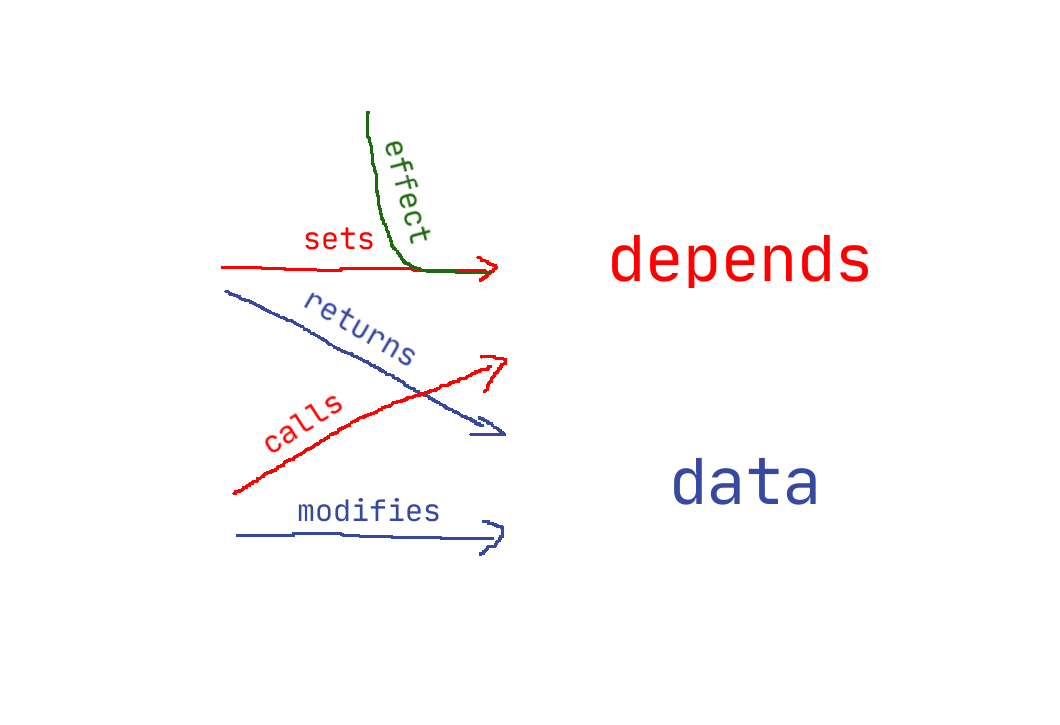### Part 1b - reactivity (all in one)

This tutorial aims to explain how reactivity is implemented in Vue3. Part 1b is meant to be more of a “quick lookup” then in depth tutorial, if you want that, check part 1a.

``````const data = reactive({
num1: 1,
num2: 2,
sum: 0
});

useEffect(() => {
data.sum = data.num1 + data.num2;
});

// 1 + 1 = 2
console.log(`\${data.num1} + \${data.num2} = \${data.sum}`);

data.num1 = 5;

// 5 + 1 = 6
console.log(`after change: \${data.num1} + \${data.num2} = \${data.sum}`);

data.num2 = 7;

// 5 + 7 = 12
console.log(`finally: \${data.num1} + \${data.num2} = \${data.sum}`);
``````

First of all, we wrap our values with `reactive` and then use `useEffect` to make `sum` auto update.### `reactive`

At the core of this reactivity implementation lie Proxy and Reflect.

`Proxy` is a little known class that allows you to modify how you interact with objects, while `Reflect` fixes all (most?) the stuff related to `this`.

``````const reactive = (target) => {
const depends = {};
return new Proxy(target, {
if (currentEffect) {
// this part can be replaced with fancy stuff like WeakMap / Set
if (!depends[key])
depends[key] = new Set();
}
},
const ret = Reflect.set(obj, key, value, receiver);
if (depends[key])
depends[key].forEach(cb => cb());
return ret;
}
});
}
``````

### `useEffect`

This is a wrapper for our effects which simply sets and unsets `currentEffect`, so that we know if property was modified during our effect or something else did it in the meantime.

``````const useEffect = (effect) => {
currentEffect = target;
target();
currentEffect = null;
}
``````